# Introductory examples¶

Introductory examples for time series in general.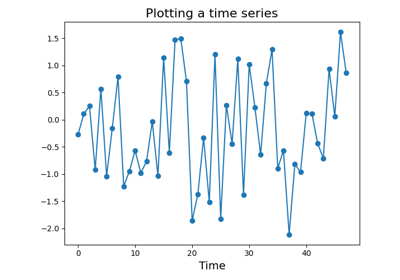Plotting a time series

# Approximating time series¶

Approximation algorithms try to capture the most important information from time series. They can be seen as simple feature extraction algorithms.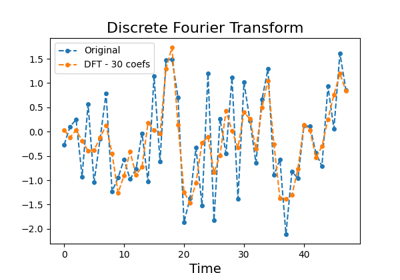Discrete Fourier Transform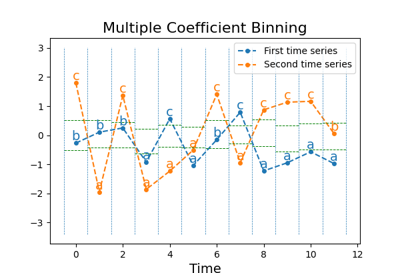Multiple Coefficient Binning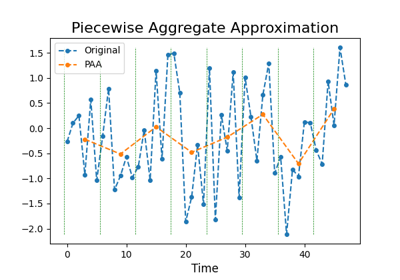Piecewise Aggregate Approximation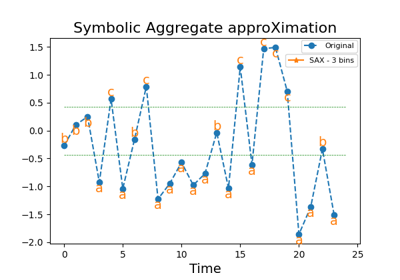Symbolic Aggregate approXimation

# Bag-of-words transformation¶

Bag-of-words algorithms transform a sequence of symbols into a bag of words.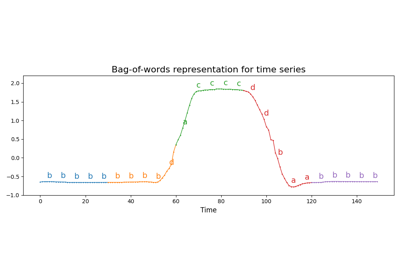Bag of Words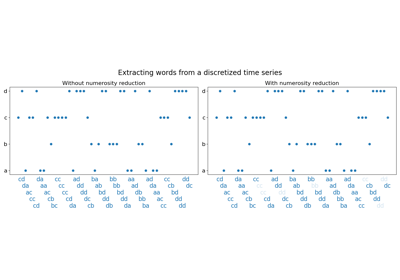Word Extractor

# Classification algorithms¶

Classification algorithms can directly classify raw time series.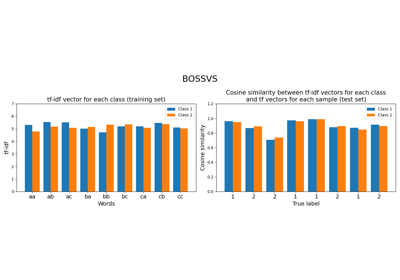Bag-of-SFA Symbols in Vector Space (BOSSVS)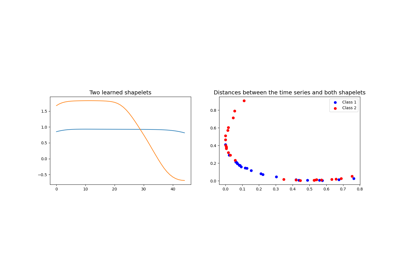Learning Time-Series Shapelets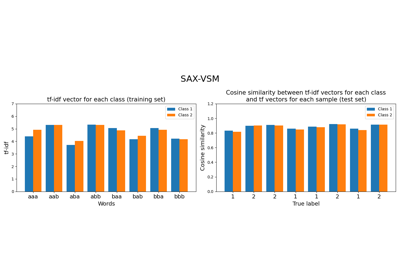Symbolic Aggregate approXimation in Vector Space Model (SAX-VSM)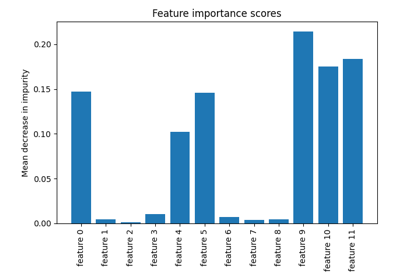Time Series Bag-of-Features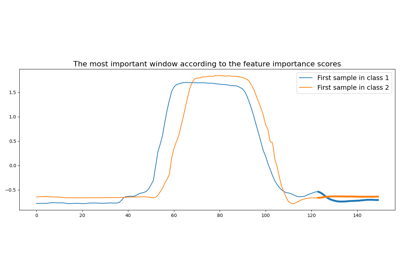Time Series Forest

# Dataset utilities¶

Examples on how to load and make time series datasets.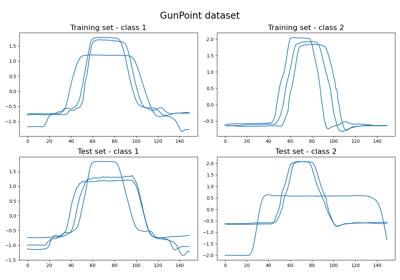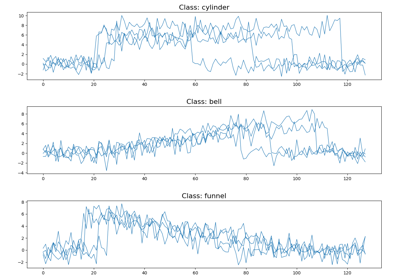Making a Cylinder-Bell-Funnel dataset

# Decomposing time series¶

Decomposition algorithms decompose time series into several time series.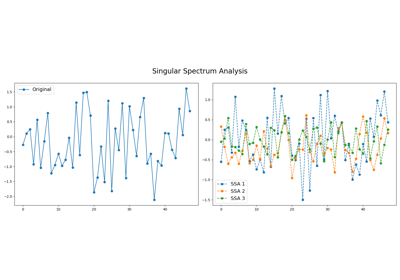Singular Spectrum Analysis

# Imaging time series¶

Imaging algorithms transform time series into images.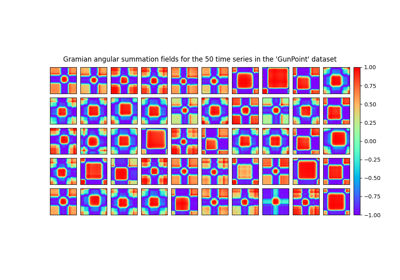Data set of Gramian angular fields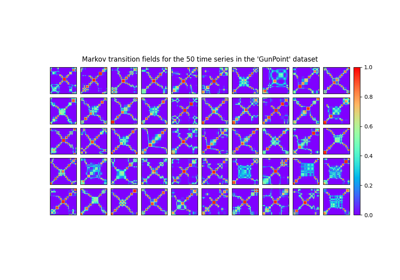Data set of Markov transition fields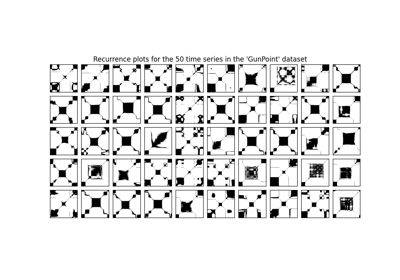Data set of recurrence plots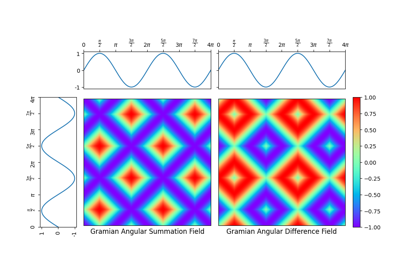Single Gramian angular field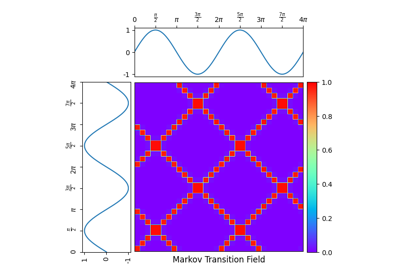Single Markov transition field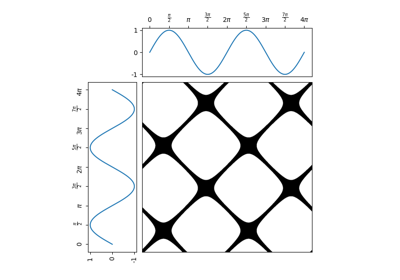Single recurrence plot

# Metrics¶

Specific metrics for time series have been developed. The examples below illustrate some of the implemented metrics.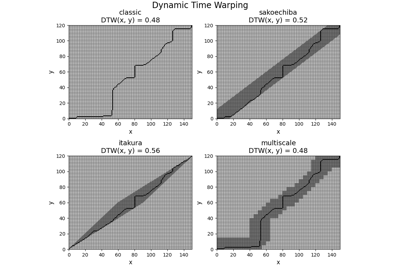Dynamic Time Warping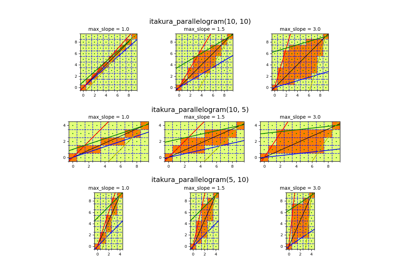Itakura parallelogram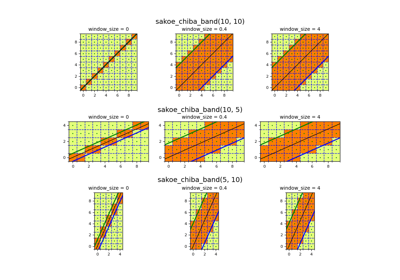Sakoe-Chiba band

# Multivariate time series¶

Specific algorithms for multivariate time series have been developed. The examples below illustrate some of the implemented ones.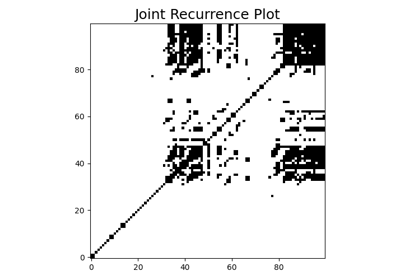Joint Recurrence Plot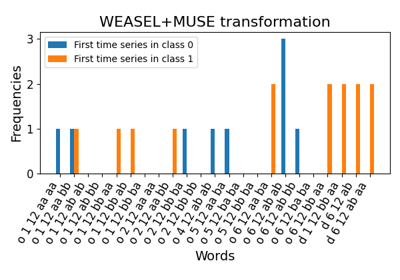WEASEL+MUSE

# Preprocessing tools¶

Preprocessing data is a common task in machine learning. The examples below illustrate the preprocessing tools available in this module.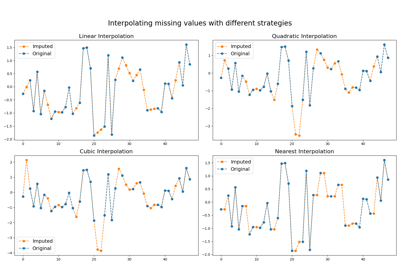Imputer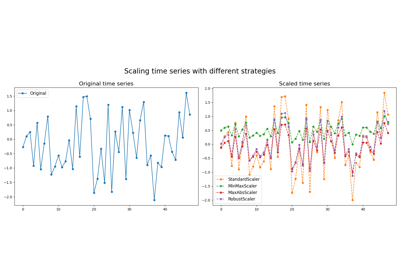Scalers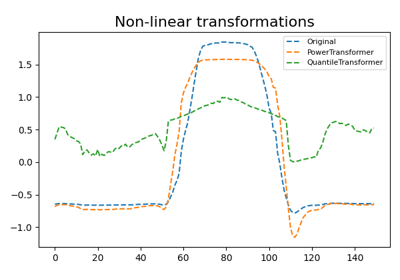Transformers

# Transformation algorithms¶

Transformation algorithms try to capture the most important information from time series using advanced transformation. They can be seen as complex feature extraction algorithms.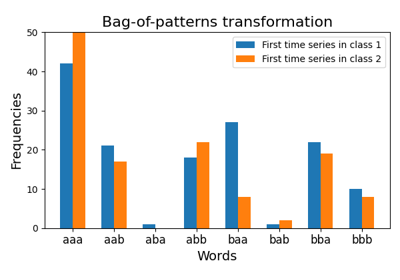Bag of Patterns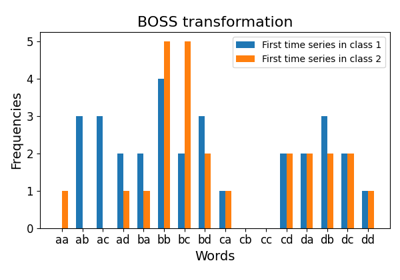Bag-of-SFA Symbols (BOSS)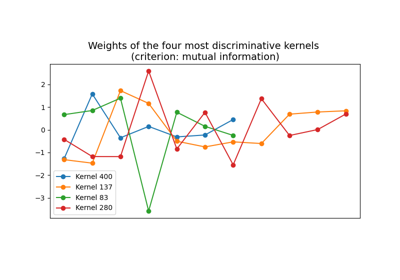RandOm Convolutional KErnel Transform (ROCKET)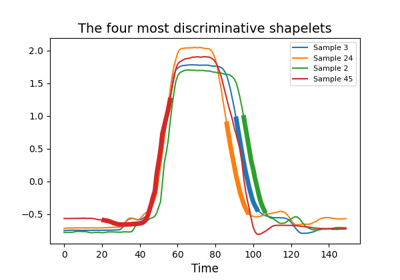Shapelet Transform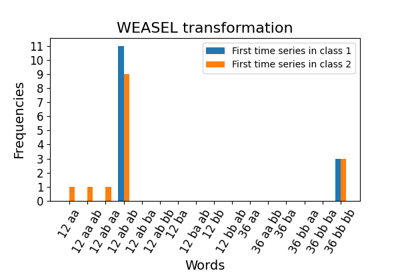Word ExtrAction for time SEries cLassification (WEASEL)

Gallery generated by Sphinx-Gallery